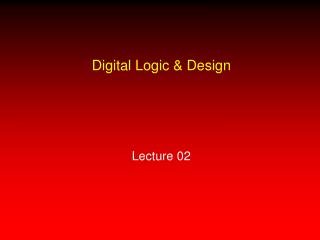DownloadDownload PresentationDigital Logic & Design Lecture 02

# Digital Logic & Design Lecture 02

Download Presentation## Digital Logic & Design Lecture 02

- - - - - - - - - - - - - - - - - - - - - - - - - - - E N D - - - - - - - - - - - - - - - - - - - - - - - - - - -
##### Presentation Transcript

1. Digital Logic & Design Lecture 02

2. Recap Last lecture discussion • Decimal Number Systems • Caveman Base 5 Number System • Binary Number System • Number System Conversion Today’s lecture discussion • Decimal-Binary Conversion

3. Binary to Decimal Conversion • Sum-of-Weights • Expression base number & weights • Sum terms • Paper and pencil method • Sum of non-zero terms • Mental Arithmetic, quick method • Sum of weights of non-zero terms

4. Binary to Decimal Conversion • Sum-of-Weights • 100112 • (1 x 24) + (0 x 23) + (0 x 22) + (1 x 21) + (1 x 20) • Terms 16, 0, 0, 2 and 1 • 19

5. Binary to Decimal Conversion • Add weights of non-zero terms • Weights increase/decrease by power of 2 • 100112 = 16 + 2 + 1 = 19 • 1011.1012 = 8 + 2 + 1 + 1/2 + 1/8 = 11 + 5/8 = 11.625

6. Decimal to Binary Conversion • Sum-of-Weights method used in reverse • Highest binary weight less than the decimal number • Subsequent smaller weights that add up to decimal number • Repeated division by 2 • Paper and pencil method • Number repeatedly divided by 2

7. Decimal to Binary Conversion using Sum-of-Weights

8. Decimal to Binary Conversion

9. Binary-Decimal fraction conversion • Binary to Decimal Conversion • Sum-of-Weights method • Weights decrease by a factor of 2 • 0.11012 weights ½, ¼, 1/16 • Sum up to 0.8125 • Decimal to Binary Conversion • Repeated Multiplication by 2 • example

10. Decimal-Binary fraction conversion • Decimal to Binary Conversion • Repeated multiplication by 2

11. Binary Arithmetic • Binary Addition • Binary Subtraction • Binary Multiplication • Binary Division

14. Binary Subtraction • Four Basic rules for binary subtraction

15. Binary Subtraction

16. Binary Multiplication • Four Basic rules for binary multiplication • Example of Binary Multiplication

17. Binary Multiplication 1101 (13) x 101 (5) 1st product term 1101 2nd product term 0000 3rd product term 1101 Product 1000001 (65)

18. Multiplication by shifting left • Decimal 29 shifted left by one digit • 290 • Shift left 1 digit is multiply by 10 • Binary 111012 (29) shifted left by one bit • 1110102 (58) • Shift left 1 bit is multiply by 2

19. Binary Division 10 101 | 1101 101 011 000 11

20. Division by shifting right • Decimal 29 shifted right by one digit • 2.9 • Shift left 1 digit is divide by 10 • Binary 111012 (29) shifted left by one bit • 1110.12 (14.5) • Shift left 1 bit is divide by 2

21. Signed and Unsigned Numbers • Unsigned Binary Numbers • Signed Binary Numbers • Most significant bit represents sign • 0 represents a positive number • 1 represents a negative number

22. 2’s Complement form • 1’s complement form • 2’s complement form Binary number 01101 (13) 1’s complement 10010 + 1 2’s complement 10011 (-13)

23. Addition and Subtraction with 2’s Complement 0101 +5 0101 +5 0010 +21110 -2 0111 +7 0011 +3 1011 -5 1011 -5 1110 -20010 +2 1001 -7 1101 -3

24. Range of Numbers • Maximum Range • Number of digits • Decimal number example • Binary number example • Overflow

25. Range of Binary Numbers

26. Range of Binary Numbers

27. Summary • Binary to Decimal Conversion • Sum-of-Weights • Sum of non-zero terms • Decimal to Binary Conversion • Sum-of-Weights (in reverse) • Repeated Division by 2

28. Summary • Binary to Decimal fraction conversion • Sum-of-Weights • Binary to Decimal fraction conversion • Repeated Multiplication by 2

29. Summary • Binary Addition • Binary Subtraction • Binary Multiplication • Multiplication by shift left operation • Binary Division • Division by shift right operation

30. Summary • Unsigned Binary • Signed Binary • Sign Bit • 2’s Complement • 1’s Complement • Range of Binary Numbers

31. Lecture No. 2 Number Systems